Different formats can be applied to the numbers that are contained in the cells. The formats affect how the numbers are displayed. For example, numbers can be represented as units of currency, percentages, fractions, time, or date. Some formats allow to configure how negative numbers are represented, divide groups of digits in numbers, and select the number of digits displayed after the decimal point.

Number formats are important for correctly interpreting data in application operations, such as calculations in formulas.

## Identify and modify cell format

 By default, all cells are assigned the General format when documents are created. To modify the format, do as follows: 1.Select the required cell/range of cells or rows/columns you want to change the format of. 2.Select the desired format from the drop-down list. On the Toolbar, in the Number section, click on the field that displays the name of the current format, and select the desired format from the drop-down list.

## General

 The General format is assigned to all cells by default when the sheet is created. The General format is universal, that is, it can be used in all formulas and functions. The following numbers in the General format are automatically displayed in scientific notation: •Prime numbers consisting of more than 11 characters. •Fractional numbers in which the integer part consists of more than 11 characters. Once this numbers are entered in the cell, they are displayed in scientific notation. For fractional numbers in the General format, non-significant zeros in the fractional part are not displayed.

## Number

 The main entry format for numbers. The following parameters can be set for the Number format: •Displaying numbers with a thousands separator. •The number of characters displayed after the dot (.) delimiter. •Negative number presentation type. These parameters can be set using the number format setting window. You can also set the number of characters displayed after the dot (.) delimiter using the buttons on the Toolbar.

## Currency

 The Currency format is used to display numbers with a currency sign or code, which corresponds to the system settings of your computer. The currency sign used by default can be modified.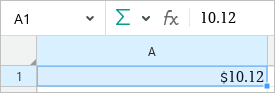A number formatted as Currency To quickly convert a cell into the Currency format once the data is entered, perform the following actions: 1.Select a cell or a range the format of which you want to change. 2.On the Toolbar, select the Number section and click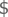Currency. A cell is automatically formatted as Currency if you specify the currency code next to the number. For example, 23.00 USD or USD 23.00. For the full list of supported currencies and their codes, see Appendix B. If a cell is formatted as Text, entering the currency code will not affect the cell format. The following parameters can be set for the number in the Currency format: •Currency code and symbol to be entered next to the number. •The number of characters displayed after the dot (.) delimiter. •Negative number presentation type. These parameters can be set using the number format setting window. You can also set the number of characters displayed after the dot (.) delimiter using the buttons on the Toolbar.

## Accounting

 The Accounting cell format is applied to numbers used in accounting documents. In the Accounting format, the entered number is automatically supplemented with the currency symbol which corresponds to the system settings of your computer. When you enter numbers in the Accounting format: •0 (zero) in cells is automatically replaced with a hyphen (-). •Negative numbers in cells are put in parentheses. In the Formula bar and in the editing mode, zeros and negative numbers are displayed the way they were entered.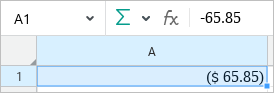A negative number formatted as Accounting For the number in the Accounting format, you can enter the currency code or symbol displayed next to the number and specify the number of decimal places displayed after the dot (.) delimiter. These parameters can be set using the number format setting window. You can also set the number of characters displayed after the dot (.) delimiter using the buttons on the Toolbar.

## Date, Time, and Date and Time

If you enter a number in the Date, Time or Date and Time format in a cell, the cell is automatically assigned the Date, Time or Date and Time format respectively.

If necessary, date and/or time display format can be changed using the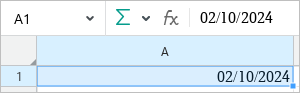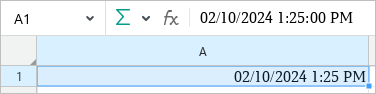A number formatted as Date A number formatted as Time A number formatted as Date and Time

Using formats in calculations

The original number corresponds to all data in the Date and Time formats. Its starting point for all dates is December 30, 1899, whose original number is 0. For example, the original number for the date of January 1, 1900 is 2.

The original number for data in the Date format will always be integer. To see this number, change the cell format to General or Number.

The original number for data in the Time format will always be a number from the 0.0 to 0.999988426 range. To see this number, change the cell format to Number.

The original numbers are required in calculations. Otherwise cells with dates would be considered as text cells and could not act as arguments in formulas and functions.

## Percentage

 The Percentage format is used to display numbers as percentages. When applying the Percentage format, the entered number is multiplied by 100 and designated with a percent sign (%). The initial value is displayed in the Formula bar.A number formatted as Percentage To convert a cell into the Percentage format quickly, perform the following actions: 1.Select a cell or a range the format of which you want to change. 2.On the Toolbar, select the Number section and click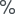Percentage. For the numbers in the Percentage format, you can set the number of characters displayed after the dot (.) delimiter using the number format setting window or the buttons on the Toolbar.

## Fraction

 This format is used to display fractional numbers as ordinary fractions, that is, the fractional part is replaced by a numerator and denominator.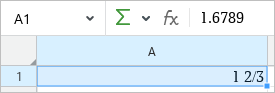A number formatted as Fraction In the Fraction format, the numerator and denominator are calculated in two ways: •Exact (for example, 0.5 = 1/2). •Approximately (for example, 1.6789 = 1 2/3).

## Scientific

 The Scientific format is used to display large numbers in a short form. In the Scientific format, part of the entered number is replaced by E + n, where E denotes the scientific notation (the preceding number is multiplied by 10 to the power of n). For example, in the Scientific format the mass of the planet Earth (5,980,000,000,000,000,000,000,000 kg) is represented as 5.98E+24, which means 5.98 multiplied by 10 to the power of twenty-four. If the Scientific format is applied to a cell, the number in the cell is displayed in the scientific notation regardless of how many characters it contains. At the same time, numbers that contain up to and including 15 characters are displayed in their original form in the edit mode and in the Formula bar. A number in the Scientific format is displayed in a cell as follows: •The integer part, always consisting of one digit. •The delimiter separating the integer and the fractional parts. •The fractional part, which by default consists of two digits. The number of digits in the fractional part can be decreased or increased as needed. •The power of 10 specified as E.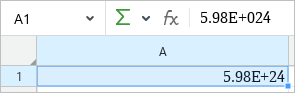A number formatted as Scientific

## Text

 In the Text format, the entered data is not transformed and is displayed in the same way in a cell and in the Formula bar. Compared to the General format, data in the Text format is not used in the calculations, even if there is a number inside the cell. This format is useful when you want to exclude some numbers from an argument array.

## Number format settings

 You can customize the display of data in cells for which the format Number, Currency, Accounting, Date and/or Time, and Percentage is selected. To customize the formats, follow these steps: 1.Select the cell/range or rows/columns where you want to customize the data display. 2.Open the number format settings window in one of the following ways: •In the Format menu, select Number format. •On the Toolbar, select the Number section, display the list of available formats and select Advanced settings. •Right-click the selected cells or the titles/contents of the selected rows/columns to open the context menu and select Number format in the context menu. 3.In the Number format window, select the format you want to customize from the list to the left. 4.Specify the parameters for the format (see the description below). 5.Click ОК. To navigate through the Number format window, use the Tab button (to move from left to right) or the Shift+Tab combination (to move from right to left). If a list is selected, use the ↓ and ↑ buttons to navigate through it. Number format settings You can set the following parameters for the Number format: •Use thousands separator: If this box is checked, groups of digits in numbers are separated by a comma. For example, the number 123456.00 is displayed as 123,456.00. •Decimal places: With this option you can increase or decrease the number of decimal places. You can also increase and decrease the number of decimal places using the buttons on the Toolbar. •Negative numbers: Select the way negative numbers formatted as Number are displayed. Currency format settings You can set the following parameters for the Currency format: •Symbol: Currency code or symbol used next to the numbers formatted as Currency. •Decimal places: This option allows you to increase or decrease the number of decimal places. You can also increase and decrease the number of decimal places using the buttons on the Toolbar. •Negative numbers: Select the way negative numbers formatted as Currency are displayed. Accounting format settings You can set the following parameters for the Accounting format: •Symbol: Currency code or symbol used next to the numbers formatted as Accounting. •Decimal places: This option allows you to increase or decrease the number of decimal places. You can also increase and decrease the number of decimal places using the buttons on the Toolbar. Date, Time, and Date and Time format settings You can set the following parameters for the cells formatted as Date, Time, and Date and Time: •To display the Date, select the format from the Date list. In the Time list, select None. •To display the Time, in the Date list select None. In the Time list, select the format you want to apply. •To display Date and Time, select the formats you want to use both in the Date and Time lists. Percentage format settings •Decimal places: This option allows you to increase or decrease the number of decimal places. You can also increase and decrease the number of decimal places using the buttons on the Toolbar.

## Increase or decrease decimals

 For the numbers in the Number, Currency, Accounting, Percentage, and Scientific formats it is possible to increase or decrease the number of characters displayed after the delimiter. When decreasing the decimals, the last visible decimal is automatically rounded up or down depending on the nearest value. Standard number rounding rules are applied. You can adjust the number of decimals displayed after the dot (.) delimiter using the following tools: •Number format settings window (not available for the Scientific format) •Toolbar buttons To increase or decrease the number of characters after the dot (.) delimiter using the buttons on the Toolbar, follow the steps below: 1.Select a cell or a cell range containing the numbers you want to modify. 2.To increase the number of characters after the delimiter, on the Toolbar, select the Number section and click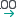Increase Decimals. To decrease the number of characters after the delimiter, clickDecrease Decimals.

## Text formatting of formulas and numbers

 In some cases, a formula or number that is entered into a cell needs to be retained in its original form. For example, display zero characters when entering the number 00056 or specify a formula without its further use. To do this, the cell with the number or formula should be formatted as Text. The formatting is applied by using a single quotation mark ('). The single quotation mark should be entered in a cell immediately before the formula or number to be recognized as text. For example: '=SUM(B1;C1) or '00056. If the single quotation mark is set in front of the data in a cell, then by pressing the Enter key the cell format changes automatically to Text. The single quotation mark is not displayed in the cell or in the Formula bar. The Text formatting is supported in all spreadsheet applications. However, in some third-party applications the format of cells may remain General as set by default. If a document contains such cells, then the first time you open the document in the MyOffice Spreadsheet application, their format changes automatically to Text. The following message appears on the screen: "Cells that contain data beginning with a single quotation mark (') were formatted as Text". Click Close on this message. To change the Text format of a cell to another format (for example, to apply a formula), perform the actions described in the Identify and modify cell format section.

Was the material useful?
Yes
No